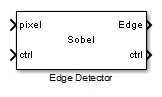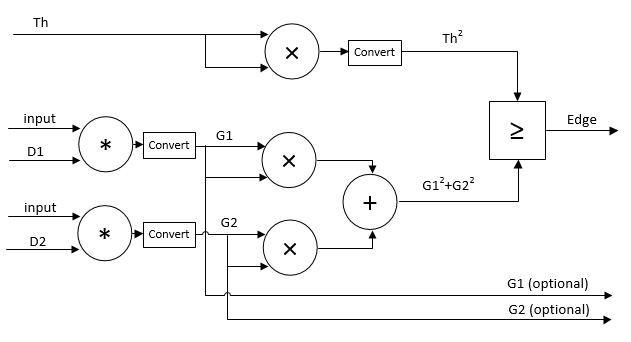# Edge Detector

Find edges of objects in grayscale pixel stream

• Library:
• Vision HDL Toolbox / Analysis & Enhancement

•## Description

The Edge Detector block finds the edges in a grayscale pixel stream by using the Sobel, Prewitt, or Roberts method. The block convolves the input pixels with derivative approximation matrices to find the gradient of pixel magnitude along two orthogonal directions. It then compares the sum of the squares of the gradients to the square of a configurable threshold to determine if the gradients represent an edge.

By default, the block returns a binary image as a stream of pixel values. A pixel value of 1 indicates that the pixel is an edge. You can disable the edge output. You can also enable output of the gradient values in the two orthogonal directions at each pixel.

## Ports

This block uses a streaming pixel interface with a bus for frame control signals. This interface enables the block to operate independently of image size and format. The pixel, Edge, and gradient ports on this block support single pixel streaming or multipixel streaming. Single pixel streaming accepts and returns a single pixel value each clock cycle. Multipixel streaming accepts and returns a vector of 4 or 8 pixels per clock cycle to support high-frame-rate or high-resolution formats. Along with the pixel, the block accepts and returns a `pixelcontrol` bus containing five control signals. The control signals indicate the validity of each pixel and their location in the frame. For multipixel streaming, one set of control signals applies to all four or eight pixels in the vector. To convert a frame (pixel matrix) into a serial pixel stream and control signals, use the Frame To Pixels block. For a full description of the interface, see Streaming Pixel Interface.

### Input

expand all

This block supports single pixel streaming or multipixel streaming. For single pixel streaming, specify a single input pixel as a scalar intensity value. For multipixel streaming, specify a vector of four or eight pixel intensity values. For details of how to set up your model for multipixel streaming, see Filter Multipixel Video Streams.

`double` and `single` data types are supported for simulation, but not for HDL code generation.

Data Types: `uint8` | `uint16` | `uint32` | `int8` | `int16` | `int32` | `fixed point` | `Boolean` | `double` | `single`

Control signals associated with the pixel stream, specified as a `pixelcontrol` bus that contains five signals. The signals describe the validity of the pixel and its location in the frame. For more information, see Pixel Control Bus.

For multipixel streaming, each vector of pixel values has one set of control signals. Because the vector has only one `valid` signal, the pixels in the vector must be either all valid or all invalid. The `hStart` and `vStart` signals apply to the pixel with the lowest index in the vector. The `hEnd` and `vEnd` signals apply to the pixel with the highest index in the vector.

Data Types: `bus`

Threshold value that defines an edge, specified as a scalar. The block compares the square of this value to the sum of the squares of the gradients.

`double` and `single` data types are supported for simulation, but not for HDL code generation.

Data Types: `single` | `double` | `int8` | `int16` | `int32` | `uint8` | `uint16` | `uint32` | `fixed point`

### Output

expand all

For single pixel streaming, Edge is a Boolean scalar. For multipixel streaming, Edge is a vector of NumberOfPixels-by-1 Boolean values. NumberOfPixels can be four or eight. Each pixel value indicates whether the pixel is an edge.

Data Types: `Boolean`

Vertical and horizontal gradient values calculated over the kernel centered at a pixel location.

For single pixel streaming, the block returns Gv and Gh as scalar values. For multipixel streaming, the block returns Gv and Gh as vectors of NumberOfPixels-by-1 values. NumberOfPixels can be four or eight.

`double` and `single` data types are supported for simulation, but not for HDL code generation.

#### Dependencies

These ports are visible when you set Method to `Sobel` or `Prewitt`.

Data Types: `single` | `double` | `int8` | `int16` | `int32` | `uint8` | `uint16` | `uint32` | `fixed point`

Orthogonal gradient values calculated over the kernel centered at a pixel location.

For single pixel streaming, the block returns G45 and G135 as scalar values. For multipixel streaming, the block returns G45 and G135 as vectors of NumberOfPixels-by-1 values. NumberOfPixels can be four or eight.

`double` and `single` data types are supported for simulation, but not for HDL code generation.

#### Dependencies

These ports are visible when you set Method to `Roberts`.

Data Types: `single` | `double` | `int8` | `int16` | `int32` | `uint8` | `uint16` | `uint32` | `fixed point`

Control signals associated with the pixel stream, specified as a `pixelcontrol` bus that contains five signals. The signals describe the validity of the pixel and its location in the frame. For more information, see Pixel Control Bus.

For multipixel streaming, each vector of pixel values has one set of control signals. Because the vector has only one `valid` signal, the pixels in the vector are either all valid or all invalid. The `hStart` and `vStart` signals apply to the pixel with the lowest index in the vector. The `hEnd` and `vEnd` signals apply to the pixel with the highest index in the vector.

Data Types: `bus`

## Parameters

expand all

### Main

When you select `Sobel` or `Prewitt`, the block calculates horizontal and vertical gradients, `Gv` and `Gh`. When you select `Roberts`, the block calculates orthogonal gradients, `G45` and `G135`. For details of each method, see Algorithms.

### Note

If you select `Prewitt`, the full-precision internal data type is large due to the 1/6 coefficient. Consider selecting Output the gradient components, so that you can customize the data type to a smaller size.

When this parameter is selected, the block returns a stream of binary pixels representing the edges detected in the input frame.

You must select at least one of Output the binary image and Output the gradient components.

When this parameter is selected, the block returns a stream of values representing the gradients calculated in the two orthogonal directions at each pixel. When you set Method to `Sobel` or `Prewitt`, the output ports Gv and Gh appear on the block. When you set Method to `Roberts`, the output ports G45 and G135 appear on the block.

You must select at least one of Output the binary image and Output the gradient components.

You can set the threshold from an input port or from the dialog box. The default value is `Property`. Selecting `Input port` enables the Th port.

The block compares the square of this value to the sum of the squares of the gradients. The block casts this value to the data type of the gradients.

#### Dependencies

This option is visible when you set Source of threshold value to `Property`.

Specify a power of two that accommodates the number of active pixels in a single horizontal line.

If you specify a value that is not a power of two, the block uses the next largest power of two. The block allocates (N – 1)-by-Line buffer size memory locations to store the pixels, where N is the number of lines in the differential approximation matrix. If you set Method to `Sobel` or `Prewitt`, then N is 3. If you set Method to `Roberts`, then N is 2.

• `Symmetric` — Set the value of the padding pixels to mirror the edge of the image. This option prevents edges from being detected at the boundaries of the active frame.

• `None` — Exclude padding logic. The block does not set the pixels outside the image frame to any particular value. This option reduces the hardware resources used by the block and the blanking required between frames but affects the accuracy of the output pixels at the edges of the frame. To maintain pixel stream timing, the output frame is the same size as the input frame. However, to avoid using pixels calculated from undefined padding values, mask off the KernelSize/2 pixels around the edge of the frame for downstream operations. For details, see Increase Throughput with Padding None.

### Data Types

Specify a rounding method for internal fixed-point calculations.

When you clear this parameter, fixed-point and integer values wrap around to zero when the value overflows what is representable with that data type. When you select this parameter, the value saturates at the maximum representable value.

Data type for the two gradient output ports. By default, the block automatically chooses full-precision data types.

#### Dependencies

To enable this parameter, on the Main tab, select Output the gradient components.

## Algorithms

expand all

The Edge Detector block provides three methods for detecting edges in an input image. The methods use different derivative approximation matrices to find two orthogonal gradients. The Sobel and Prewitt methods calculate the gradient in horizontal and vertical directions. The Roberts method calculates the gradients at 45 degrees and 135 degrees. The block uses the same matrices as the Edge Detection block in Computer Vision Toolbox™.

When you use multipixel streaming, the block uses a single line memory and implements NumberOfPixels filters in parallel. This increase in hardware resources is a trade off for increasing throughput compared to single-pixel streaming.

MethodDirection 1Direction 2
Sobel$\frac{1}{8}\left[\begin{array}{ccc}1& 0& -1\\ 2& 0& -2\\ 1& 0& -1\end{array}\right]$$\frac{1}{8}\left[\begin{array}{ccc}1& 2& 1\\ 0& 0& 0\\ -1& -2& -1\end{array}\right]$
Prewitt$\frac{1}{6}\left[\begin{array}{ccc}1& 0& -1\\ 1& 0& -1\\ 1& 0& -1\end{array}\right]$$\frac{1}{6}\left[\begin{array}{ccc}1& 1& 1\\ 0& 0& 0\\ -1& -1& -1\end{array}\right]$
Roberts$\frac{1}{2}\left[\begin{array}{cc}1& 0\\ 0& -1\end{array}\right]$$\frac{1}{2}\left[\begin{array}{cc}0& 1\\ -1& 0\end{array}\right]$

### Note

The Prewitt coefficients require extra bits of precision because they are not powers of two. The block uses 16 bits to represent the Prewitt coefficients. For 8-bit input, the default size of the full-precision gradients is 27 bits. When using the Prewitt method, a good practice is to reduce the word length used for the gradient calculation. Select the Output the gradient components check box, and then on the Data Types tab, specify a smaller word length using Gradient Data Type.

The block convolves the neighborhood of the input pixel with the derivative matrices, D1 and D2. It then compares the sum of the squares of the gradients to the square of the threshold. Computing the square of the threshold avoids constructing a square root circuit. The block casts the gradients to the type you specified on the Data Types tab. The type conversion on the square of the threshold matches the type of the sum of the squares of the gradients.## Extended Capabilities

### Objects

#### Introduced in R2015a

##### SupportGet trial now